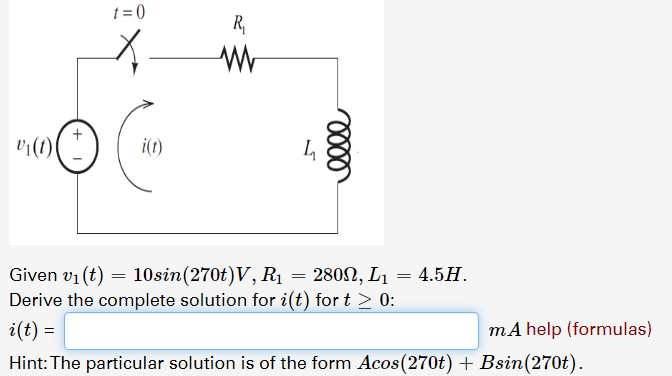# v()Given v1 (t) 10sin(270t) V, RiDerive the complete solution for i(t) for t> 02802, L1 4.5HmA help (formulas)i(t)Hint: The particular solution is of the form Acos(270t) + Bsin(270t).

Question

Textbook Problemhelp_outlineImage Transcriptionclosev() Given v1 (t) 10sin(270t) V, Ri Derive the complete solution for i(t) for t> 0 2802, L1 4.5H mA help (formulas) i(t) Hint: The particular solution is of the form Acos(270t) + Bsin(270t). fullscreen
check_circleExpert Solution
Step 1

The given circuit is a series R-L circuit. Expression of current is as follows,

Step 2

Steady-state current is equal to the particular solution of the differential equation.

The transient current is equal to the complementary solution of the differential equation.

Step 3

The solution is of...

### Want to see the full answer?

See Solution

#### Want to see this answer and more?

Solutions are written by subject experts who are available 24/7. Questions are typically answered within 1 hour*

See Solution
*Response times may vary by subject and question
Tagged in

### Electrical Engineering